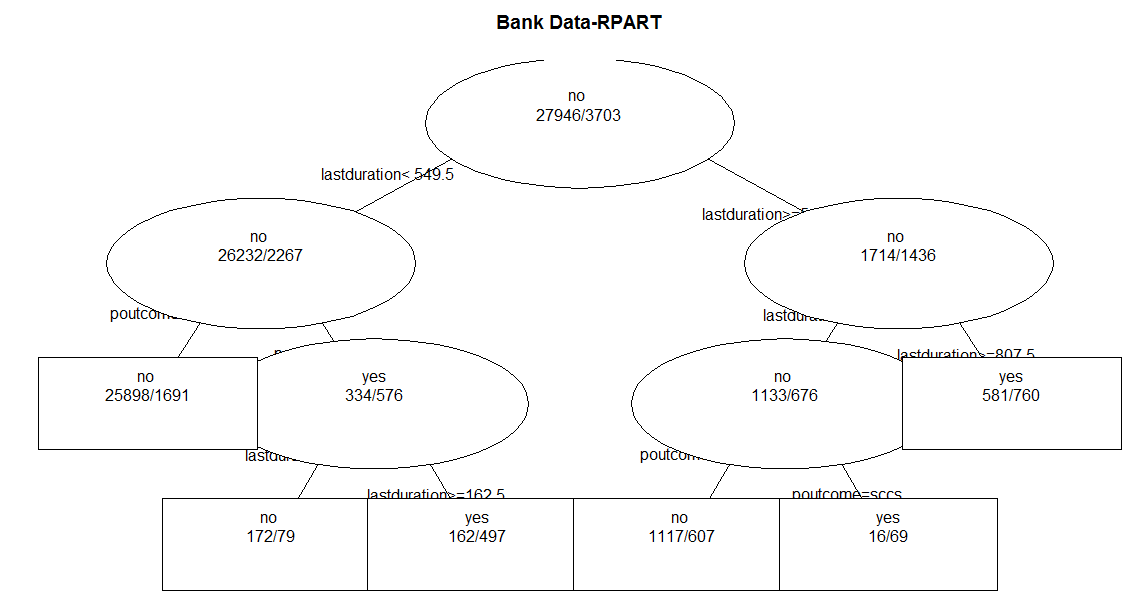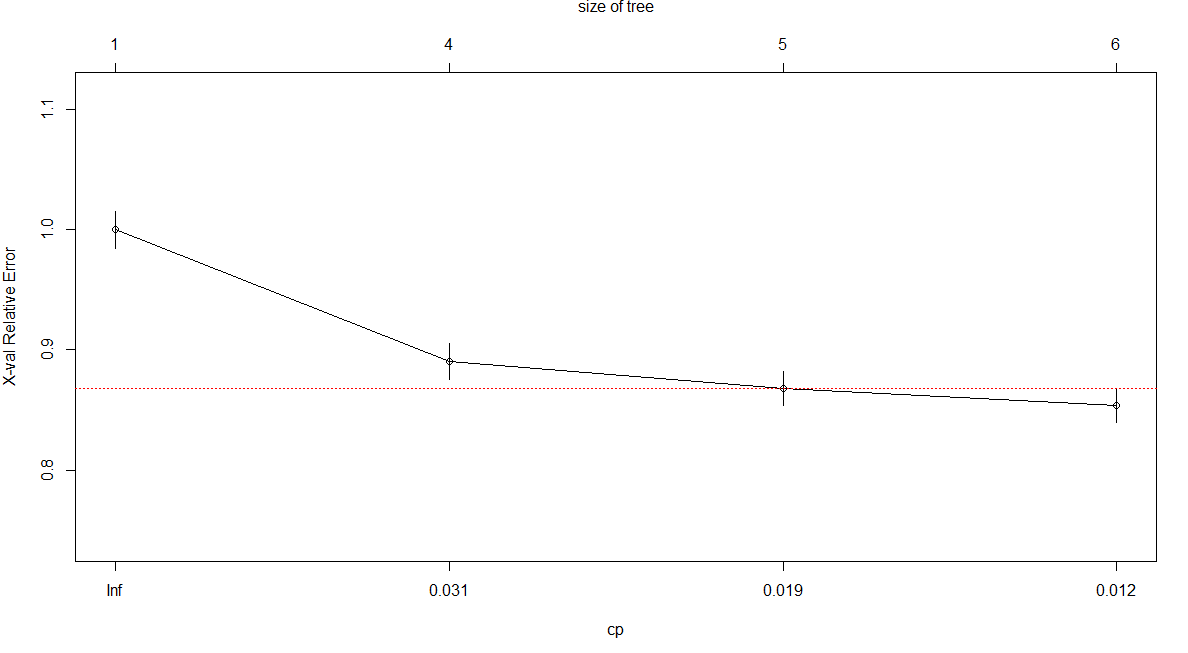## Wednesday, 7 May 2014

### R Code - Bank Subscription Marketing - Classification {RECURSIVE PARTITIONING AND REGRESSION TREES}

R Code  for Recursive Partitioning and Regression Trees (RPART)
Data Set:- Bank Marketing https://www.blogger.com/blogger.g?blogID=4359514443959080595#editor/target=post;postID=5426750358330739357
Source of Data Set:-  UCI Repository (http://archive.ics.uci.edu/ml/datasets/Bank+Marketing)
The Code includes the following:-
1. Data Exploration - Missing Values, Outliers
2. Data Visualisation
3.  Correlation Matrix
4. Data Partitioning
5. Package used "rpart"
6. Model Tuning and Plots
7. Confusion Matrix

## The data has been imported using Import Dataset option in R Environment
## The data set can be obtained from http://archive.ics.uci.edu/ml/datasets/Bank+Marketing
## DATASET UNDERSTANDING
head(bank_full) ## Displays first 6 rows for each variable
str(bank_full) ## Describes each variables
summary(bank_full) ## Provides basic statistical information of each variable

## DATA EXPLORATION - Check for Missing Data
## Option 1
is.na(bank_full) ## Displays True for a missing value
## Since it is a large dataset, graphical display of missing values will prove to be easier
##Option 2
require(Amelia)
missmap(bank_full,main="Missing Data - Bank Subscription", col=c("red","grey"),legend=FALSE)

## No red colour stripes are visible. hence no missing values.
##Option 3
summary(bank_full) ## displays missing values if any under every variable

## DATA VISUALISATION
## Use Box plots (Only for continuous variables)- To Check Ouliers
boxplot(bank_full\$age~bank_full\$subscribed, main=" AGE",ylab="age of customers",xlab="Subscribed")
boxplot(bank_full\$balance~bank_full\$subscribed, main=" BALANCE",ylab="Balance of customers",xlab="Subscribed")
boxplot(bank_full\$lastday~bank_full\$subscribed, main=" LAST DAY",ylab="Last day of contact",xlab="Subscribed")
boxplot(bank_full\$lastduration~bank_full\$subscribed, main="LAST DURATION",ylab="Last duration of contact",xlab="Subscribed")
boxplot(bank_full\$numcontacts~bank_full\$subscribed, main="NUM CONTACTS",ylab="number of contacts",xlab="Subscribed")
boxplot(bank_full\$pdays~bank_full\$subscribed, main=" Previous DAYS",ylab="Previous days of contact",xlab="Subscribed")
boxplot(bank_full\$pcontacts~bank_full\$subscribed, main=" Previous Contacts",ylab="Previous Contacts with customers",xlab="Subscribed")

## Though some outliers are observed in Previous contacts, NumContacts and LastDuration, they have not bee removed keeping their significance into consideration
## Use Histograms (For both continuous and categorical variables)
## These histograms provide details abpout Skewness, Normal Distribution etc
## Function to create histograms for continuous variables with normal curve
bank_Conthist<-function(VarName,NumBreaks,xlab,main,lengthxfit) ## xlab and main should be mentioned under quotes as they are characters
{
hist(VarName,breaks=NumBreaks,col="yellow",xlab=xlab,main=main)
xfit<-seq(min(VarName),max(VarName),length=lengthxfit)
yfit<-dnorm(xfit,mean=mean(VarName),sd=sd(VarName))
yfit<-yfit*diff(h\$mids[1:2])*length(VarName)
lines(xfit,yfit,col="red",lwd=3)
}
bank_Conthist(bank_full\$age,10,"age of customers","AGE",30)
bank_Conthist(bank_full\$balance,50,"Balance of customers","Balance",100)

## Balance is more skewed towards to Negative or Zero
bank_Conthist(bank_full\$lastday,5,"Last Day of contact","LAst Day",10)
bank_Conthist(bank_full\$lastduration,100,"LastDuration of COntact","Last Duration",10)

## Last Duration is more skewed towards 0 to 100 secs.
bank_Conthist(bank_full\$numcontacts,30,"Number of Contacts","NUmContacts",20)
## NUmContacts are more skewed towards 1
bank_Conthist(bank_full\$pdays,30,"Previous Days of contacts","PDays",20)
## Many were not contacted previously
bank_Conthist(bank_full\$pcontacts,20,"Previous Contacts","PContacts",10)
## Since many were not contacted previously, therefore Pcontacts is 0

## Barplots for Categorical Variables
barplot(table(bank_full\$job),col="red",main="JOB")
barplot(table(bank_full\$marital),col="green",main="Marital")
barplot(table(bank_full\$education),col="red",main="Education")
barplot(table(bank_full\$creditdefault),col="red",main="Credit Default")

## Since Credit Default is highly skewed towards NO, this shall be removed from further analysis
bank_full<-NULL
str(bank_full)
barplot(table(bank_full\$housingloan),col="red",main="Housing Loan")
barplot(table(bank_full\$personalloan),col="blue",main="Personal Loan")
barplot(table(bank_full\$lastcommtype),col="red",main="Last communication type")
barplot(table(bank_full\$lastmonth),col="violet",main="Last Month")
barplot(table(bank_full\$poutcome),col="magenta",main="Previous Outcome")

## Correlation Matrix among input (or independent) continuous variables
bank_full.cont<-data.frame(bank_full\$age,bank_full\$balance,bank_full\$lastday,bank_full\$lastduration,bank_full\$numcontacts,bank_full\$pdays,bank_full\$pcontacts)
str(bank_full.cont)
cor(bank_full.cont)

## It can be observed that No two variables are highly correlated

## Partitioning Data into Train and Test datasets in 70:30
library(caret)
set.seed(1234567)
train2<-createDataPartition(bank_full\$subscribed,p=0.7,list=FALSE)
train<-bank_full[train2,]
test<-bank_full[-train2,]
write.table(bank_full,"bank1.csv",sep=",")
str(train)
str(test)

## CLASSIFICATION USING Recursive partitioning and regressive trees(RPART) Model

install.packages("rpart")
library(rpart)

## Various parameters that control resursive partioning and regressive trees
control<-rpart.control(minsplit=30,minbucket=10,cp=0.01,maxcomplete=6,maxsurrogate=8,usersurrogate=2,xval=15,surrogatestyle=0,maxdepth=15)
## generally minimum no. of observations in any terminal leaf node(minbucket)=minimum no. of observations that must exist in a node in order to split (minsplit)/3
##Training the model
train.rpart<-rpart(subscribed~.,data=train,control=control)
##Prints the rpart object
print(train.rpart)
##Summarizes the model
summary(train.rpart,digits=getOption("digits"),cp=0,save="train.rpart")
## MEan Variance Plot- Graphical representation of deviance of a node divided by the number of observations at the node
meanvar(train.rpart)  ## Drawn for Regression plots..Not significant for Classification plots
##Shows paths to the selected nodes- It returns the names list where each element contains the splits on the path from the root to the selected node
path.rpart(train.rpart,nodes=c(10,11,12,13),pretty=0,print.it=TRUE)
##Plot the rpart object
plot(train.rpart,uniform=FALSE,branch=1,compress=TRUE,margin=0)
##Label the plot drawn above (TREE Dendogram)
text(train.rpart,splits=TRUE,use.n=TRUE)
##PostScript Presentation Plot
post(train.rpart,file="",title="Bank Data-RPART",use.n=TRUE)
##Snip-Contains the nodes that remain after selected subtrees are snipped off
train.rpart.snip<-snip.rpart(train.rpart,toss=5)  ## trim subtree at node=5
plot(train.rpart.snip)
text(train.rpart.snip,splits=TRUE,use.n=TRUE)
post(train.rpart.snip,file="",title="Bank Data-RPART",use.n=TRUE)

## R squared plots for different splits
rsq.rpart(train.rpart)
## Prediction on TEST data set using Trained model
##Prediction with Class Probabilities
test.rpart.prob<-predict(train.rpart,test,type="prob")
test.rpart.prob

## Level Numbers- Predicted
test.rpart.vector<-predict(train.rpart,test,type="vector")
test.rpart.vector

## Factors - Predicted
test.rpart.factor<-predict(train.rpart,test,type="class")
test.rpart.factor

## Matrix of Level numbers, class frequencies, and probabilities
test.rpart.matrix<-predict(train.rpart,test,type="matrix")
test.rpart.matrix

## Misclassification table or Confusion Matrix
table(test.rpart.vector,test\$subscribed)
## Residuals from a fitted rpart
residuals(train.rpart,type=c("usual","pearson","deviance"))  ## This has more significance in Regression type dataset
##Predicted values after cross vaildation for a set of complexity parameter values
##cp is desired list of complexity values. By default takes values from cptable component fit
##Displays cptable
printcp(train.rpart)
train.rpart.cv<-xpred.rpart(train.rpart,xval=15,cp=4)

## Find MSE (Mean squared Error) with cross-validated model- Cross Validated Error estimate
## More valid for regression analysis
train.error<-(train.rpart.cv-train\$subscribed)^2
apply(train.error,2,sum)

## Visual representation of cross validation results-Plots a complexity parameter table
plotcp(train.rpart,minline=TRUE,lty=3,col="red",upper=c("size","splits","none"))
##Cost complexity pruning-Determines nested sequence of subtrees by recursively snipping off least important splits
prune(train.rpart,cp=4)

RPART TREEVISUAL REPRESENTATION OF CROSS VALIDATION RESULTS